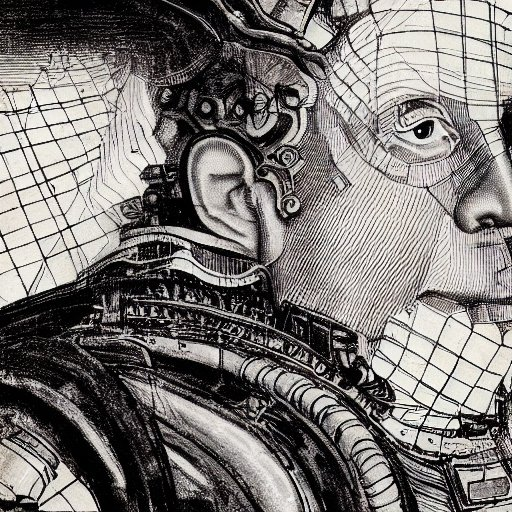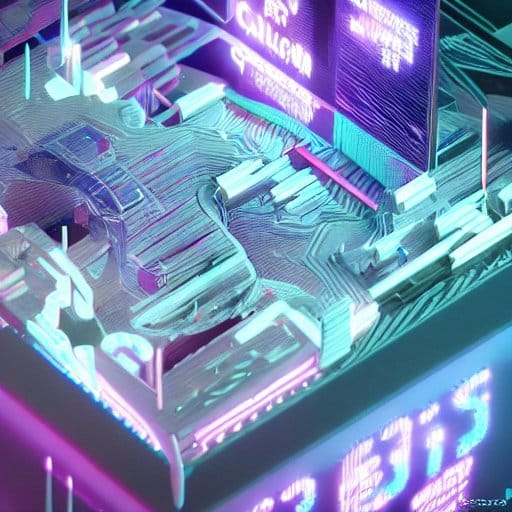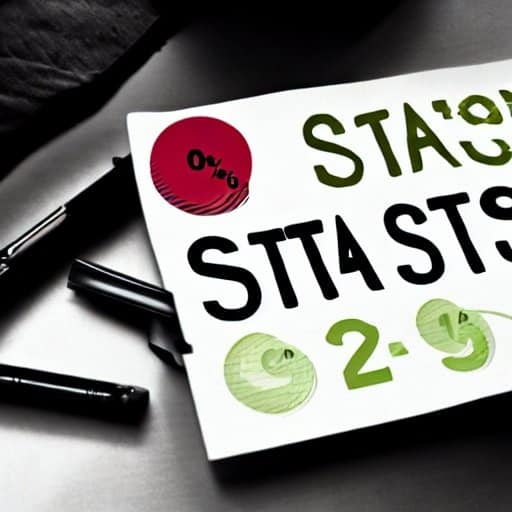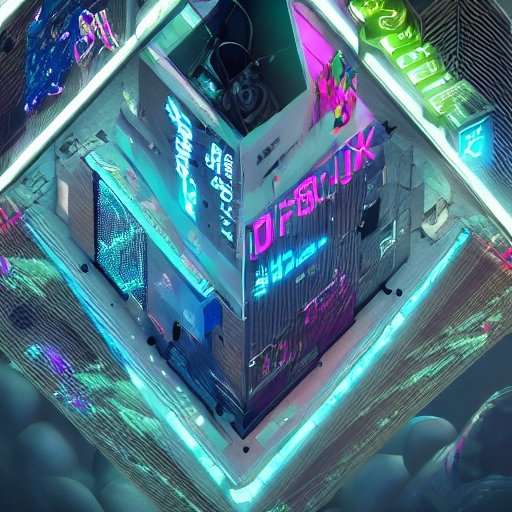Programming and mathematics have often been intertwined, leading to the common belief that programmers need to have a strong foundation in math. However, this notion has also been challenged by some who argue that math is not a prerequisite for becoming a successful programmer. In this blog post, we will delve into the relationship between programming and math, exploring the importance of math in programming and examining its practical applications. By the end, we hope to shed light on whether or not programmers truly need math skills to excel in their field.

## Why the Debate?

The debate on whether programmers need math skills stems from the diverse nature of programming itself. Programming encompasses various domains, including web development, software engineering, data analysis, and machine learning. While some programming tasks heavily rely on mathematical concepts, others may not require advanced math knowledge at all. This has led to differing opinions on the necessity of math skills for programmers.

## The Perception of Math in Programming

Mathematics has long been associated with intelligence and problem-solving skills. Therefore, many people believe that having a strong grasp of math will automatically make someone a better programmer. However, it is essential to differentiate between the ability to solve complex mathematical problems and the ability to write efficient code.

## The Role of Logic and Problem-Solving

One of the fundamental skills required in programming is logical thinking and problem-solving. Programmers need to be able to break down complex problems into smaller, manageable tasks and devise logical solutions for each step. While math can help develop these skills, it is not the only way to acquire them. Logic and problem-solving skills can be honed through practice and experience in programming itself.

## The Importance of Math in Programming

Although math may not be a mandatory requirement for all programmers, it undoubtedly plays a significant role in certain areas of programming. Understanding mathematical concepts can enhance a programmer’s ability to tackle specific challenges and optimize their code for efficiency. Let’s explore some of the key mathematical concepts that programmers may encounter in their work.

## The Relationship Between Programming and Math

The relationship between programming and math is a complex one. While not all programming tasks require advanced math skills, there are areas where a solid foundation in mathematics can greatly benefit programmers. In this section, we will explore the connection between programming and math, examining how mathematical concepts and principles can be applied in the world of programming.

### Mathematical Thinking in Programming

Mathematical thinking involves logical reasoning, problem-solving, and an ability to break down complex problems into smaller, more manageable parts. These skills are also essential in programming. In fact, many of the principles and techniques used in math, such as algorithms and formulas, are directly applicable to programming.Programmers often encounter situations where they need to analyze data, manipulate variables, or optimize code performance. These tasks require logical thinking and problem-solving abilities, which are fundamental to both math and programming. By leveraging mathematical thinking, programmers can approach problems in a structured and systematic manner, leading to more efficient and elegant solutions.

### Overlapping Concepts

There are several areas where programming and math overlap, creating a strong synergy between the two disciplines. Some of the key overlapping concepts include:

#### 1. Algorithms

Algorithms are step-by-step procedures used to solve problems. They form the foundation of both math and programming. In mathematics, algorithms are used to solve equations, find patterns, and perform calculations. In programming, algorithms are used to design efficient and optimized code, solve complex problems, and perform data manipulation.

#### 2. Data Structures

Data structures are used to organize and store data efficiently. They are vital in both math and programming. In mathematics, data structures like matrices, vectors, and graphs are used to represent and manipulate mathematical objects. In programming, data structures such as arrays, linked lists, and trees are used to store and manipulate data, enabling efficient data processing and retrieval.#### 3. Logic and Boolean Algebra

Logic and Boolean algebra play a crucial role in both math and programming. In mathematics, logic is used to prove theorems, reason mathematically, and construct valid arguments. In programming, logic and Boolean algebra are used to make decisions, control program flow, and implement conditional statements.

#### 4. Computational Mathematics

Computational mathematics involves using computers to solve mathematical problems. It combines mathematical principles with programming techniques. Computational mathematics is used in various fields, including scientific simulations, cryptography, and optimization problems. Programmers with a strong mathematical background can leverage computational mathematics to develop efficient algorithms and solve complex mathematical problems.

### Mathematical Foundations for Specialized Fields

Certain domains within programming heavily rely on mathematical foundations. For example:

#### 1. Machine Learning and Artificial Intelligence

Machine learning and artificial intelligence require a solid understanding of mathematical concepts such as statistics, linear algebra, calculus, and probability theory. These mathematical foundations enable programmers to develop and train models, analyze data, and make predictions or decisions based on patterns and statistical inferences.#### 2. Cryptography

Cryptography involves securing information and communication systems. It relies on mathematical principles like number theory, modular arithmetic, and algebraic structures. Cryptographic algorithms, such as RSA and AES, are based on mathematical concepts. Programmers working in the field of cryptography need a strong mathematical background to design and implement secure cryptographic systems.

#### 3. Computer Graphics and Game Development

Computer graphics and game development rely on concepts from linear algebra, geometry, and trigonometry. These mathematical principles are used to model and manipulate 3D objects, calculate lighting and shading effects, and simulate realistic physics in virtual environments. Programmers working in these fields need a solid understanding of these mathematical concepts to create visually stunning and immersive experiences.## Mathematical Concepts in Programming

Mathematical concepts play a vital role in programming, providing programmers with powerful tools to solve problems, optimize algorithms, and analyze data. In this section, we will explore some of the key mathematical concepts that programmers may encounter in their work.

### 1. Algebra

Algebra is a branch of mathematics that deals with symbols and the rules for manipulating these symbols. In programming, algebraic concepts are frequently used for data manipulation and problem-solving. Some areas where algebra is applied in programming include:

• Equations and Inequalities: Algebraic equations and inequalities are used to model various real-world problems in programming. They are utilized in optimization algorithms, constraint-based programming, and mathematical modeling.
• Linear Algebra: Linear algebra is extensively used in machine learning, computer graphics, and scientific computing. Concepts such as vectors, matrices, and matrix operations are essential for tasks like image processing, data transformation, and solving systems of linear equations.
• Polynomials: Polynomials are used in many programming applications, such as curve fitting, signal processing, and cryptography. They provide a mathematical representation for complex patterns and functions.### 2. Calculus

Calculus is a branch of mathematics that deals with rates of change and accumulation. Although not all programming tasks require calculus, it is widely used in fields like physics simulations, optimization problems, and data analysis. Some key concepts in calculus that are relevant to programming are:

• Derivatives: Derivatives are used to determine rates of change and gradients in functions. They are crucial in optimizing algorithms, performing numerical approximations, and solving optimization problems.
• Integration: Integration is used to calculate areas, volumes, and accumulations. It plays a significant role in tasks such as numerical integration, statistical analysis, and simulations.
• Differential Equations: Differential equations are used to model dynamic systems and phenomena. They are widely used in simulations, physics engines, and scientific computations.

### 3. Probability and Statistics

Probability and statistics are essential for data analysis, machine learning, and decision-making in programming. Understanding these concepts enables programmers to work with uncertainty, make predictions, and draw meaningful conclusions from data. Some key concepts in probability and statistics include:

• Probability Theory: Probability theory is used to model and analyze random events and uncertainty. It is fundamental to tasks such as statistical modeling, hypothesis testing, and Monte Carlo simulations.
• Descriptive Statistics: Descriptive statistics involves summarizing and visualizing data using measures such as mean, median, variance, and correlation. These statistics provide insights into data distributions and patterns.
• Inferential Statistics: Inferential statistics is used to make predictions and draw conclusions about populations based on sample data. It is essential for tasks like hypothesis testing, regression analysis, and confidence interval estimation.### 4. Discrete Mathematics

Discrete mathematics deals with mathematical structures that are fundamentally discrete rather than continuous. It forms the basis for many programming concepts and algorithms. Some key areas of discrete mathematics that are relevant to programming include:

• Graph Theory: Graph theory is used to model relationships between objects. It is employed in network analysis, social network analysis, and algorithms like Dijkstra’s algorithm and depth-first search.
• Combinatorics: Combinatorics involves counting, arranging, and selecting objects. It is used in tasks such as generating permutations, calculating probabilities, and designing efficient algorithms.
• Logic: Logic is used to reason and make deductions. It forms the foundation for Boolean algebra, propositional logic, and predicate logic, which are essential for conditional statements and decision-making in programming.

## Practical Applications of Math in Programming

Mathematics has numerous practical applications in the field of programming. From data analysis to cryptography, understanding mathematical concepts allows programmers to tackle complex problems and optimize their code. In this section, we will explore some of the practical applications of math in programming.

### 1. Data Analysis and Statistics

Data analysis is a crucial aspect of programming, and mathematics provides the necessary tools to extract meaningful insights from data. Some practical applications of math in data analysis include:

• Descriptive Statistics: Mathematical concepts such as mean, median, variance, and correlation are used to summarize and analyze data. These statistics provide insights into data distributions, relationships, and trends.
• Probability and Statistical Modeling: Probability theory and statistical models are used to analyze data and make predictions. Techniques like hypothesis testing, regression analysis, and Bayesian inference rely on mathematical principles.
• Data Visualization: Mathematics plays a vital role in creating visually appealing and informative data visualizations. Concepts like scaling, axis labeling, and color mapping are used to present data in a meaningful way.### 2. Machine Learning and Artificial Intelligence

Machine learning and artificial intelligence (AI) heavily rely on mathematical concepts and algorithms. Some practical applications of math in machine learning and AI include:

• Linear Algebra: Linear algebra is used to represent and manipulate data in machine learning models. Matrices and vectors are used to store and perform operations on large datasets.
• Calculus and Optimization: Calculus is used in optimizing machine learning algorithms. Techniques like gradient descent, which relies on derivatives, are used to find the optimal parameters of a model.
• Probability and Statistics: Probability theory and statistical models are used in machine learning for tasks like classification, clustering, and anomaly detection. These concepts help in making predictions and analyzing uncertain data.
• Neural Networks: Neural networks, a fundamental component of deep learning, are inspired by the structure and function of the human brain. They utilize mathematical concepts such as linear algebra, calculus, and probability theory for training and inference.

### 3. Cryptography and Security

Cryptography is the practice of securing information and communication systems. Mathematical principles are at the core of cryptographic algorithms. Some practical applications of math in cryptography include:

• Number Theory: Number theory is used in cryptographic algorithms like RSA (Rivest-Shamir-Adleman). Concepts such as prime numbers, modular arithmetic, and Euler’s totient function are employed to secure data.
• Discrete Logarithms: Discrete logarithms are used in cryptographic protocols like Diffie-Hellman key exchange and elliptic curve cryptography. These mathematical concepts ensure secure key generation and exchange.
• Symmetric and Asymmetric Encryption: Encryption algorithms, both symmetric and asymmetric, rely on mathematical principles. They use mathematical operations like modular arithmetic and exponentiation to transform data into a secure form.### 4. Computer Graphics and Game Development

Computer graphics and game development utilize mathematical principles to create visually stunning graphics and simulate realistic environments. Some practical applications of math in computer graphics and game development include:

• Linear Algebra: Linear algebra is used to represent and manipulate 3D objects and transformations. Matrices, vectors, and matrix operations are employed to perform calculations for rendering and animation.
• Geometry and Trigonometry: Geometry and trigonometry are used to calculate positions, angles, and distances in 3D space. These concepts enable the rendering of objects with accurate perspective and lighting effects.
• Physics Simulations: Physics simulations in games and simulations rely on mathematical models and equations. Concepts such as kinematics, Newton’s laws of motion, and collision detection are applied to create realistic physics interactions.

### 5. Optimization and Efficiency

Mathematics plays a crucial role in optimizing algorithms and improving code efficiency. Some practical applications of math in optimization and efficiency include:

• Algorithm Analysis: Mathematical analysis is used to evaluate the time and space complexity of algorithms. This analysis helps in selecting the most efficient algorithms for specific tasks.
• Big O Notation: Big O notation is a mathematical notation used to describe the performance of an algorithm. It provides a way to compare the efficiency of different algorithms and make informed choices.
• Numerical Methods: Numerical methods involve solving complex mathematical problems using approximation techniques. These methods are used in optimization algorithms, numerical integration, and solving differential equations.

## Conclusion

The question of whether programmers need math skills has been a topic of debate in the programming community. Throughout this blog post, we have explored the relationship between programming and math, delving into the importance of math in programming and examining its practical applications. By doing so, we have gained insights into the role of math in the world of programming.

While it is true that not all programming tasks require advanced math skills, it is undeniable that math plays a significant role in certain areas of programming. Mathematical thinking, problem-solving abilities, and logical reasoning are crucial skills that can be honed through math education. These skills are transferable to programming and can greatly enhance a programmer’s ability to tackle complex problems and optimize their code for efficiency.

We have seen how mathematical concepts like algebra, calculus, probability and statistics, and discrete mathematics find practical applications in programming. From data analysis to machine learning, cryptography to computer graphics, mathematics provides programmers with powerful tools to solve a wide range of problems.Moreover, specialized fields within programming, such as machine learning and artificial intelligence, heavily rely on mathematical foundations. Linear algebra, calculus, and probability theory are fundamental to algorithm design, model training, and data analysis in these domains. Programmers working in these areas would greatly benefit from a strong understanding of math.

However, it is important to note that while math is valuable in programming, it is not the sole determining factor of success. Programming requires a diverse set of skills, including logical thinking, problem-solving, creativity, and collaboration. These skills can be developed through practice and hands-on experience in programming itself.

Ultimately, the necessity of math skills for programmers depends on the specific domain and tasks at hand. While some programmers may rarely encounter advanced math concepts in their day-to-day work, others may work in fields where math is indispensable. However, even in cases where math is not directly used, the logical thinking and problem-solving skills developed through math education can still greatly benefit programmers.

In conclusion, while programmers do not necessarily need math skills in every aspect of their work, having a solid foundation in mathematics can significantly enhance their problem-solving abilities, optimize their code, and open doors to specialized fields within programming. Whether you choose to delve deep into math or focus on other aspects of programming, continuously learning and expanding your skills will undoubtedly contribute to your success as a programmer.